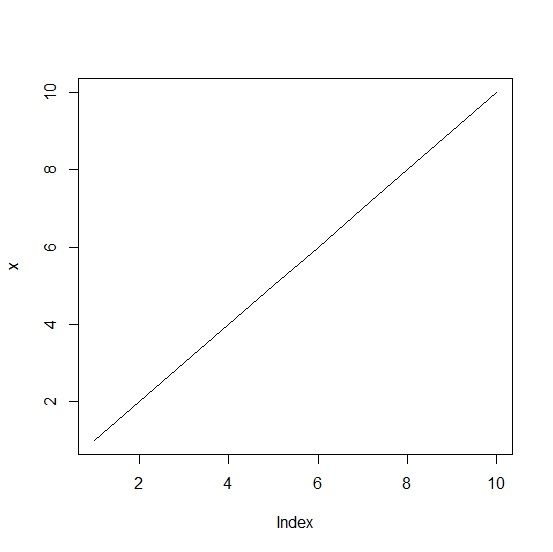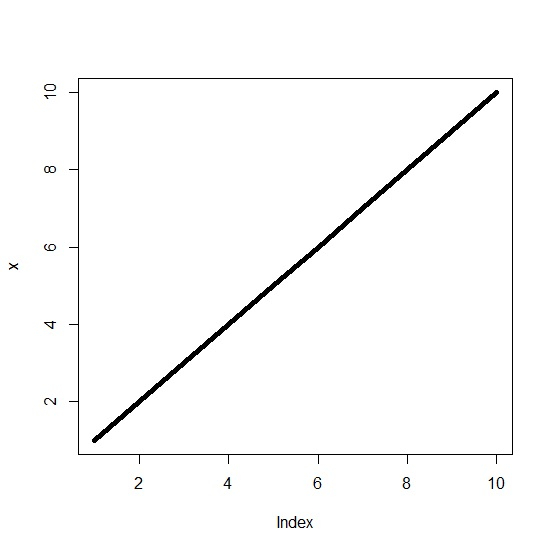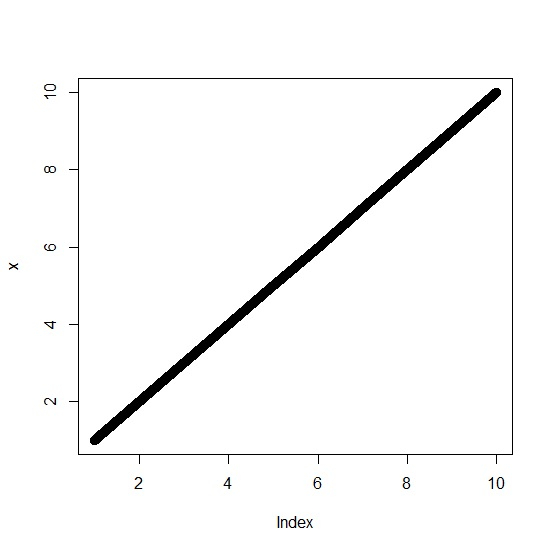# How to create a line chart in R using plot function with larger width?

To create a line chart in base R using plot function, we need to use type = "l" so that R understand the plot needs to have a line instead of points. If we want to increase the width of the line then lwd argument can be used. The value lwd = 0 is the default value for the width.

Consider the below vector and create the line chart −

## Example

x<-1:10
plot(x,type="l")

## OutputCreate the line chart with larger width of the line −

## Example

plot(x,type="l",lwd=5)

## OutputCreate the line chart with very wide line −

## Example

plot(x,type="l",lwd=10)

## Output